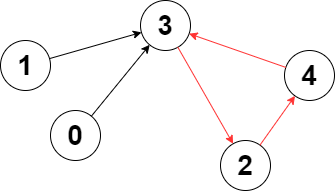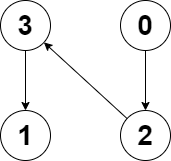##### Welcome to Subscribe On Youtube

Formatted question description: https://leetcode.ca/all/2360.html

# 2360. Longest Cycle in a Graph

• Difficulty: Hard.
• Related Topics: Depth-First Search, Graph, Topological Sort.
• Similar Questions: Strange Printer II.

## Problem

You are given a directed graph of n nodes numbered from 0 to n - 1, where each node has at most one outgoing edge.

The graph is represented with a given 0-indexed array edges of size n, indicating that there is a directed edge from node i to node edges[i]. If there is no outgoing edge from node i, then edges[i] == -1.

Return the length of the **longest cycle in the graph**. If no cycle exists, return -1.

A cycle is a path that starts and ends at the same node.

Example 1:Input: edges = [3,3,4,2,3]
Output: 3
Explanation: The longest cycle in the graph is the cycle: 2 -> 4 -> 3 -> 2.
The length of this cycle is 3, so 3 is returned.


Example 2:Input: edges = [2,-1,3,1]
Output: -1
Explanation: There are no cycles in this graph.


Constraints:

• n == edges.length

• 2 <= n <= 105

• -1 <= edges[i] < n

• edges[i] != i

## Solution

• class Solution {
public int longestCycle(int[] edges) {
int n = edges.length;
boolean[] vis = new boolean[n];
boolean[] dfsvis = new boolean[n];
int[] path = new int[n];
int maxLength = -1;
for (int i = 0; i < n; i++) {
if (!vis[i]) {
path[i] = 1;
maxLength = Math.max(maxLength, dfs(i, 1, path, vis, dfsvis, edges));
}
}
return maxLength;
}

private int dfs(
int node, int pathLength, int[] path, boolean[] vis, boolean[] dfsvis, int[] edges) {
vis[node] = true;
dfsvis[node] = true;
int length = -1;
if (edges[node] != -1 && !vis[edges[node]]) {
path[edges[node]] = pathLength + 1;
length = dfs(edges[node], pathLength + 1, path, vis, dfsvis, edges);
} else if (edges[node] != -1 && dfsvis[edges[node]]) {
length = pathLength - path[edges[node]] + 1;
}
dfsvis[node] = false;
return length;
}
}

############

class Solution {
public int longestCycle(int[] edges) {
int n = edges.length;
boolean[] vis = new boolean[n];
int ans = -1;
for (int i = 0; i < n; ++i) {
if (vis[i]) {
continue;
}
int j = i;
List<Integer> cycle = new ArrayList<>();
for (; j != -1 && !vis[j]; j = edges[j]) {
vis[j] = true;
}
if (j == -1) {
continue;
}
for (int k = 0; k < cycle.size(); ++k) {
if (cycle.get(k) == j) {
ans = Math.max(ans, cycle.size() - k);
break;
}
}
}
return ans;
}
}

• class Solution:
def longestCycle(self, edges: List[int]) -> int:
n = len(edges)
vis = [False] * n
ans = -1
for i in range(n):
if vis[i]:
continue
curr = i
cycle = []
while curr != -1 and not vis[curr]:
vis[curr] = True
cycle.append(curr)
curr = edges[curr]
if curr == -1:
continue
for j, v in enumerate(cycle):
if v == curr:
ans = max(ans, len(cycle) - j)
break
return ans

############

# 2360. Longest Cycle in a Graph
# https://leetcode.com/problems/longest-cycle-in-a-graph/

class Solution:
def longestCycle(self, edges: List[int]) -> int:
n = len(edges)
visited = [False] * n
res = -1

for node in range(n):
dist = {}
d = 0

while node != -1 and not visited[node]:
visited[node] = True
dist[node] = d
node = edges[node]
d += 1

if node != -1 and node in dist:
res = max(res, d - dist[node])

return res


• class Solution {
public:
int longestCycle(vector<int>& edges) {
int n = edges.size();
vector<bool> vis(n);
int ans = -1;
for (int i = 0; i < n; ++i) {
if (vis[i]) {
continue;
}
int j = i;
vector<int> cycle;
for (; j != -1 && !vis[j]; j = edges[j]) {
vis[j] = true;
cycle.push_back(j);
}
if (j == -1) {
continue;
}
for (int k = 0; k < cycle.size(); ++k) {
if (cycle[k] == j) {
ans = max(ans, (int) cycle.size() - k);
break;
}
}
}
return ans;
}
};

• func longestCycle(edges []int) int {
vis := make([]bool, len(edges))
ans := -1
for i := range edges {
if vis[i] {
continue
}
j := i
cycle := []int{}
for ; j != -1 && !vis[j]; j = edges[j] {
vis[j] = true
cycle = append(cycle, j)
}
if j == -1 {
continue
}
for k := range cycle {
if cycle[k] == j {
ans = max(ans, len(cycle)-k)
break
}
}
}
return ans
}

func max(a, b int) int {
if a > b {
return a
}
return b
}

• function longestCycle(edges: number[]): number {
const n = edges.length;
const vis = new Array(n).fill(false);
let ans = -1;
for (let i = 0; i < n; ++i) {
if (vis[i]) {
continue;
}
let j = i;
const cycle: number[] = [];
for (; j != -1 && !vis[j]; j = edges[j]) {
vis[j] = true;
cycle.push(j);
}
if (j == -1) {
continue;
}
for (let k = 0; k < cycle.length; ++k) {
if (cycle[k] == j) {
ans = Math.max(ans, cycle.length - k);
break;
}
}
}
return ans;
}



Explain:

nope.

Complexity:

• Time complexity : O(n).
• Space complexity : O(n).